Next Continued Fraction Arithmetic 34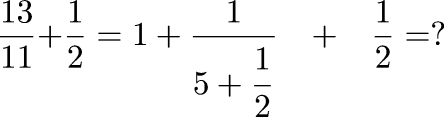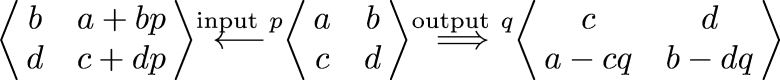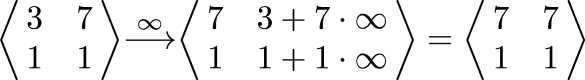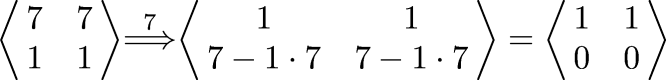• z has so far output [1; 1, 2, 7] = 1 + 1/(1 + 1/(2 + 1/7))

• = 1 + 1/(1 + 7/15)

• = 1 + 15/22

• = 37/22

• This is indeed the correct answer (we were computing 13/11 + 1/2, remember)

• What if we ask z for another term?

• It knows that its own value is 1/0 =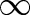• So it reports that it has no more terms left

 Next Back